## Find the force of gravity between a newborn baby (mass = 2.9 kg ) and planet Mars (mass = 6.4×1023kg) when Mars is at its closest to Earth (

Question

Find the force of gravity between a newborn baby (mass = 2.9 kg ) and planet Mars (mass = 6.4×1023kg) when Mars is at its closest to Earth (distance = 5.6×1010m ).

in progress 0
2 weeks 2021-08-28T18:41:02+00:00 1 Answers 0 views 0

The force of gravity between the newborn baby and Mars is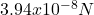Explanation:

The universal law of gravitation is defined as: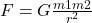(1)

Where G is the gravitational constant, m1 and m2 are the masses of the two objects and r is the distance between them.

Since the newborn baby is at the Earth, it will be at a distance (r) of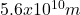from Mars.

Therefore, equation 1 can be used: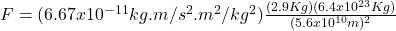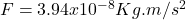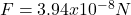Hence, the force of gravity between the newborn baby and Mars is.